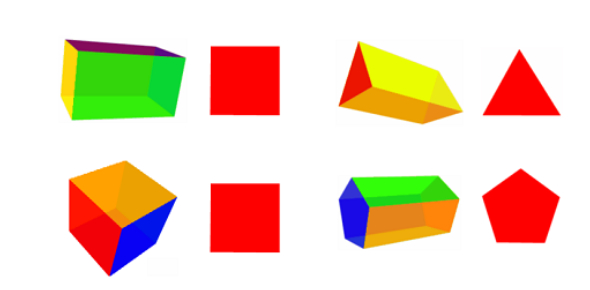# Identify The Basic 3D Shapes: Quiz!

10 Questions | Attempts: 5524
ShareSettingsA 3D shape is represented by its edges, faces, and vertices, measured in 3 directions, known as three-dimensional shapes. The length, width, and height are the three measuring units ina 3D shape. Take this quiz to test your knowledge about different 3D shapes. All the best!

• 1.
What shape is a 3d circle?
• A.

Cube

• B.

Rectangle

• C.

Circle

• D.

Sphere

• 2.
What shape is the cross-section of the sphere?
• A.

Cone

• B.

Circle

• C.

Pyramid

• 3.
What kind of shape will you get with a cross-section parallel to the base?
• A.

Cylinder

• B.

Circle

• C.

Cube

• 4.
Will the cross-section be congruent to the base of a cylinder.
• A.

No

• B.

Yes

• 5.
What is a description of a cone?
• A.

A figure with a circle top

• B.

A figure with a pointed top and a circled bottom

• C.

A figure with a square top

• 6.
A 3-D shape can also be called...
• A.

A flat shape

• B.

A solid shape

• C.

A polygon

• D.

• 7.
Vertices are also called...
• A.

Edges

• B.

Corners

• C.

Faces

• D.

Sides

• 8.
A solid object has six faces which are all squares. What is the name of this object?
• A.

Cube

• B.

Cuboid

• C.

Cylinder

• D.

Rectangle

• 9.
Which 3-D shape has six rectangular faces?
• A.

Sphere

• B.

Cuboid

• C.

Cylinder

• D.

Square

• 10.
Most dice are the shape of which 3-D shape?
• A.

Cuboid

• B.

Cylinder

• C.

Cube

• D.

Square

## Related TopicsBack to top
×

Wait!
Here's an interesting quiz for you.